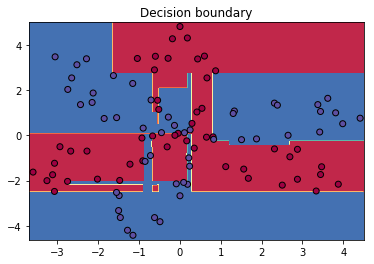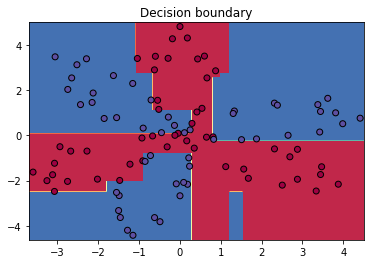### Elan Ding¶

Modified: Aug. 17, 2018 $\newcommand{\bs}{\boldsymbol}$ $\newcommand{\argmin}{\underset{\bs{#1}}{\text{arg min}}\,}$ $\newcommand{\argmax}{\underset{\bs{#1}}{\text{arg max}}\,}$ $\newcommand{\tr}{^{\top}}$ $\newcommand{\norm}{\left|\left|\,#1\,\right|\right|}$ $\newcommand{\given}{\,|\,}$

Recall from my previous post, the Forward Stagewise Additive Modeling (Algorithm 1) requires the following "greedy" minimization scheme:

$$(\beta_m, \gamma_m) = \argmin{\beta, \gamma} \sum_{i=1}^N L(r_{m-1}^{(i)}, \beta b(\bs{x}^{(i)}; \gamma)),\tag{1}$$

where $r_{m-1}^{(i)} = y^{(i)} - f_{m-1} (\bs{x}^{(i)})$ is the residual from $f_{m-1}$.

For two-class classification, the function $b(\bs{x}^{(i)}; \gamma_m) = \gamma_{j,m}$ is assumed to be a classification tree whose leaves gave outputs $\gamma_{j,m} \in \{-1, 1\}$ for $j=1,..., J_m$ where $J_m$ is the number of leaves. Using the exponential loss, we derived the AdaBoost algorithm.

Now we are ready to generalize the results. Suppose that in general, we have a tree

$$T(\bs{x}; \Theta) = \sum_{j=1}^J \gamma_j I(\bs{x} \in R_j),$$

where the parameter $\Theta = \{R_j, \gamma_j\}_{j=1}^{J}$ defines the tree's structure and the leaf values. Note that the leaf values $\gamma$ is no longer restricted by the set $\{-1, 1\}$. Hence, in the optimization process, we can drop the parameter $\beta$ in (1). Hence, the Forward Stagewise Additive Modeling, yields the following optimization subproblem in each iteration:

$$\hat{\Theta}_m = \argmin{\Theta_m} \sum_{i=1}^N L(r_{m-1}^{(i)} + T(\bs{x}^{(i)}; \Theta_m)).\tag{2}$$

In general, simple fast algorithms do not exist for solving (2); instead, we can use gradient descent to approximate the solution.

In general, if we are trying to minimize the loss function,

$$L(f) = \sum_{i=1}^N L(y^{(i)}, f(\bs{x}^{(i)})),$$

where $f(\bs{x})$ is any model, such as the sum of trees.

At the $m$th iteration of gradient descent, for each training example $(\bs{x}^{(i)}, y^{(i)})$, we compute the partial derivative evaluated at the previous model $f_{m-1}$:

$$g_{m-1}^{(i)} = \left[\partial_{f(\bs{x}^{(i)})} L(y^{(i)}, f(\bs{x}^{(i)})) \right]_{f(\bs{x}^{(i)}) = f_{m-1}(\bs{x}^{(i)})}.\tag{3}$$

Now, let's go back to (2). Instead of fitting a tree to the residuals $r_{m-1}^{(i)}$, we fit trees to the negative gradient $-g_{m-1}^{(i)}$. Why is this a good idea? Recall that the gradient value (3) is the direction in which the loss function has the fastest rate of decrease. Hence, for the fastest convergence toward the minimum, the residuals $\bs{r}=(r^{(1)},..., r^{(N)})'$ should point in the same direction as the gradient $\bs{g} = (g^{(1)},.., g^{(m)})'$. This gave rise to the Gradient Tree Boosting Algorithm.

Algorithm 1 Gradient Tree Boosting Algorithm.

• Initialize $f_0(\bs{x}) = \argmin{\gamma}\sum_{i=1}^N L(y^{(i)}, \gamma)$.
• For $m=1$ to $M$:

• For $i=1,2,..., N$ compute $$g_{m-1}^{(i)} = \left[\partial_{f(\bs{x}^{(i)})} L(y^{(i)}, f(\bs{x}^{(i)})) \right]_{f(\bs{x}^{(i)}) = f_{m-1}(\bs{x}^{(i)})}.$$

• Fit a regression tree to the netative gradients $-g_{m-1}^{(i)}$, producing leaves $R_{j,m}, j=1,..., J_m$.

• For $j=1,2,..., J_m$, compute $$\gamma_{j, m} = \argmin{\gamma} \sum_{\bs{x}^{(i)} \in R_{j, m}} L(y^{(i)}, f_{m-1}(\bs{x}^{(i)})+ \gamma).$$

• Update $f_m(\bs{x}) = f_{m-1}(\bs{x}) + \sum_{j=1}^{J_m} \gamma_{j,m} I(\bs{x}\in R_{j, m}).$

• Output $f_M(\bs{x})$.

Note that for a regression problem, if we use RSS as the loss function, then the negative gradient $-g_{m-1}^{(i)}$ is

$$-\partial_{f(\bs{x}^{(i)})} \frac{1}{2}(y^{(i)} - f(\bs{x}^{(i)}))^2 = y^{(i)} - f(\bs{x}^{(i)}).$$

This means that for squared loss, using the negative gradient is exactly the same as using the ordinary residual! For $K$-class classification, suppose that the response variable $Y$ takes values in $\mathcal{G} = \{\mathcal{G}_1,..., \mathcal{G}_K\}$. We fit $K$ regressions trees, each producing a score $f_k(\bs{x}^{(i)}), k=1,2,..., K$, and final model uses the softmax function,

\begin{aligned} f(\bs{x}^{(i)}) &= \argmax{k} p_k(\bs{x}^{(i)}) \\ &= \argmax{k} \frac{e^{f_k(\bs{x}^{(i)})}}{\sum_{l=1}^K e^{f_l(\bs{x}^{(i)})}}. \end{aligned}\tag{4}

Using the multinomial cross entropy (cross entropy between the empirical distribution and a multinomial distribution, $p_{\text{model}}$) as the the loss, we have,

\begin{aligned} L(y^{(i)}, f(\bs{x}^{(i)})) &= E_{y\sim \widehat{p}} -\log p_{\text{model}}(y) \\ &= - \sum_{j=1}^K I(y^{(i)}=\mathcal{G}_j) \log p_j(\bs{x}^{(i)}) \\ &= -\sum_{j=1}^K I(y^{(i)}=\mathcal{G}_j) f_k(\bs{x}^{(i)}) + \log\left(\sum_{l=1}^K e^{f_l(\bs{x}^{(i)})}\right). \end{aligned}\tag{5}

Each tree $f_k, k=1,2,..., K$, is fitted to its respective negative gradient given by

\begin{aligned} -g_k^{(i)} &= \partial_{f_k(\bs{x}^{(i)})} L(y^{(i)}, f(\bs{x}^{(i)})) \\ &= I(y^{(i)} = \mathcal{G}_k) - \frac{e^{f_k(\bs{x}^{(i)})}}{\sum_{l=1}^K e^{f_l(\bs{x}^{(i)})}}. \end{aligned}\tag{6}

We have the following algorithm for classification.

Algorithm 2 Gradient Boosting Classification Model.

• Fit $K$ constant models $f_{0,1}, f_{0,1}, ..., f_{0,K}$ by solving $$f_{0,k}(\bs{x}) = \argmin{\gamma}\sum_{i=1}^N L(y^{(i)}, \gamma).$$
• For $m=1$ to $M$:

• For $k=1,2,...,K$,
• For $i=1,2,...,N$ compute: $$-g_{m-1, k}^{(i)} = I(y^{(i)} = \mathcal{G}_k) - \frac{e^{f_{m-1,k}(\bs{x}^{(i)})}}{\sum_{l=1}^K e^{f_{m-1,l}(\bs{x}^{(i)})}}.$$
• Fit a regression tree against the values $-g_{m-1,k}^{(i)}$, producing leaves $R_{j, m, k}, j=1,..., J_{m,k}$.
• For $j=1,2,..., J_{m,k}$, compute $$\gamma_{j, m, k} = \argmin{\gamma} \sum_{\bs{x}^{(i)} \in R_{j, m}} L(y^{(i)}, f_{m-1}(\bs{x}^{(i)})+ \gamma).$$
• Update $f_{m,k}(\bs{x}) = f_{m-1,k}(\bs{x}) + \sum_{j=1}^{J_{m,k}} \gamma_{j,m,k} I(\bs{x}\in R_{j, m,k}).$
• Output the final prediction by $$f_M(\bs{x}) = \argmax{k} \frac{e^{f_{M,k}(\bs{x}^{(i)})}}{\sum_{l=1}^K e^{f_{M,l}(\bs{x}^{(i)})}}.$$

## Implementation¶

Using sklearn, we can easily implement the model on the same dataset we used on the previou post by simply calling

from sklearn.ensemble import GradientBoostingClassifier

gbm.fit(X,y)


The decision boundary is found to be,

In :
plot_decision_boundary(gbm, X, y)Finally, just out of curiosity, I tried a modern gradient boosting method called XGBoost. You can read the paper here.

from xgboost import XGBClassifier

xgb = XGBClassifier()
xgb.fit(X,y)


Let's visualize the decision boundary.

In :
plot_decision_boundary(xgb, X, y)Just by looking at the decision boundaries, we see that the XGBoost model captures the true shape of the distribution better than traditional boosting models, such as AdaBoost and Gradient Boosting Machine.